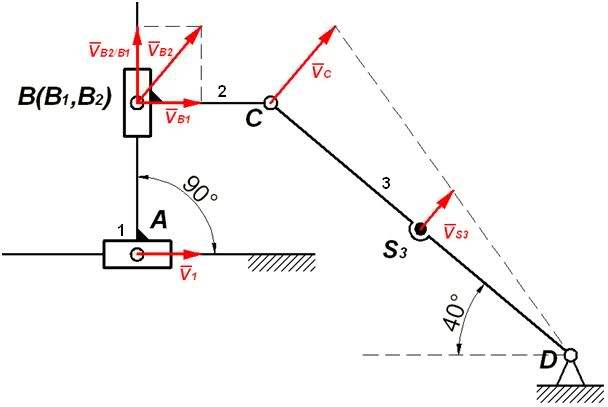# Accelerations (kinematic analysis)

Hi there;

I guess this must be really easy and obvious but I just can't seem to be able to figure it out right now. I need to find (grapho-analytically) the acceleration of B (which is obviously the same as of C) in the position show in the picture. $$v_1=2 m/s$$ is the input velocity.Data:
$$|AB|=0.068 [m], |BC|=0.06 [m], |CS_3 |=0.088 [m], |S_3 D|=0.088 [m], v_1=2 [m/s]$$

I calculated all the velocities:

$$v_{B1}=2 [m/s], v_{B2}=3.111 [m/s], v_{B2/B1}=2.384 [m/s], v_C=3.111 [m/s], v_{S3}=1.555 [m/s],$$

$$\omega_3=\frac{v_C}{|CD|}=\frac{3.111}{0.176}=17.676 [1/s]$$

As for the accelerations, certainly the acceleration of A is zero ($$v_1=const$$). Also, the normal component of the acceleration of C:

$$a_C^n=\omega_3^2\cdot{|CD|}=54.989 [m/s^2]$$

And here I am a bit lost. It looks like slider#2 is accelerating with respect to slideway#1. Any thoughts? I analyzed the structure in SAM, and the absolute acceleration of B (and C) is $$85.574 m/s^2$$. What other accelerations should I consider? Thanks in advance for taking your time.